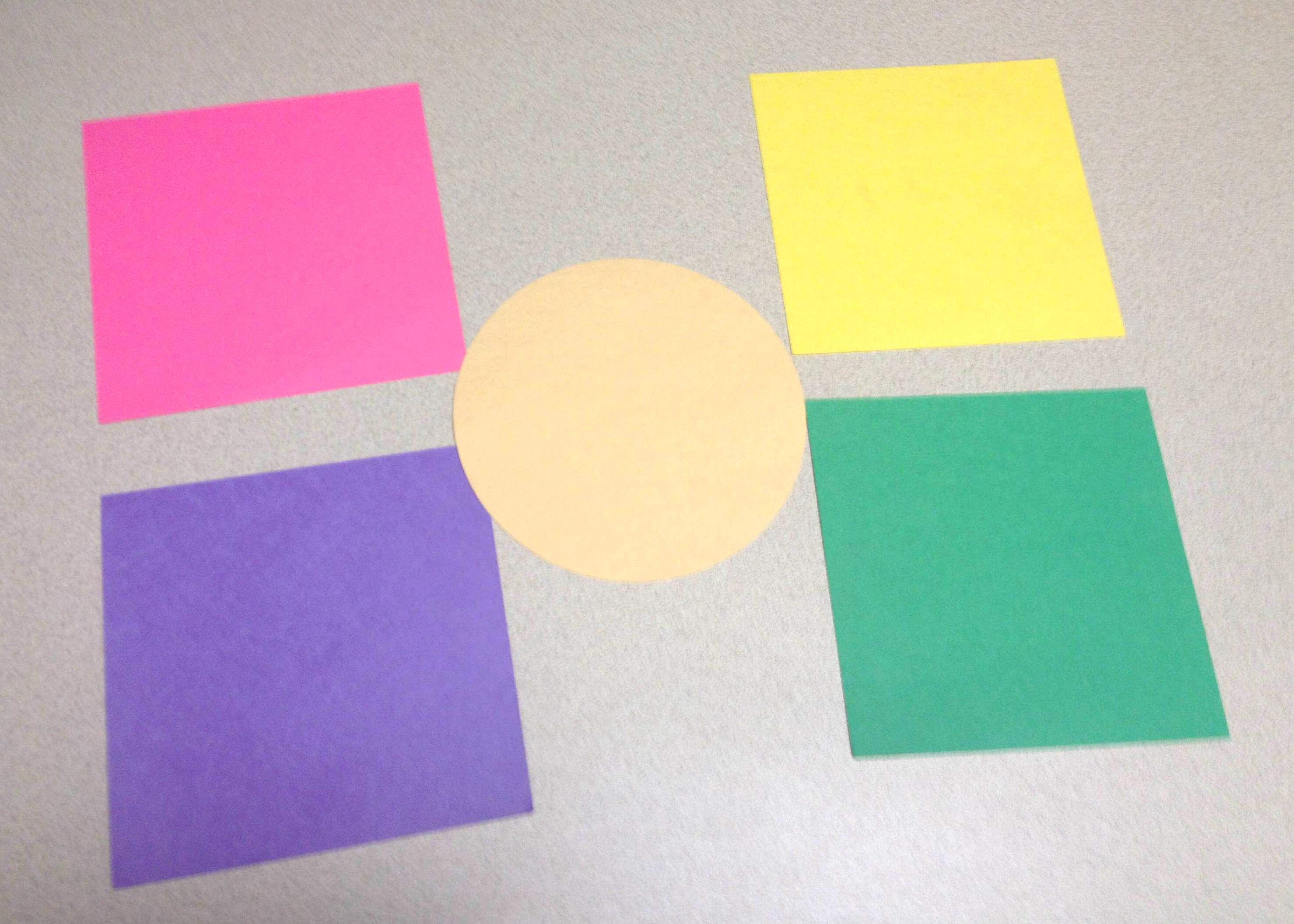April 7, 2014

Engage students with this hands-on activity that leads them to understand the formula for the area of a circle.

Pi Day, 3/14, is considered a national holiday to most math instructors. To commemorate this day, or to help incorporate a tactile learning experience in which students understand the formulas for the area and circumference of a circle, use the following paper folding activity.

Cut out circles using the Cutout Maker Circle (MTH306), at least two for each student. (This will provide students with a back up circle in case they fold their original circle incorrectly.) Cut out squares (MTH319) with side lengths equal to the radius of the circle (radius squares), four for each student. Use a different color of paper for each square so that each student receives four different colored squares.Provide each student with one circle and four different colored radius squares: The side length of each square should be equal to the radius of the circle. Give each pair of students a copy of the Explorations with a Paper Circle Activity (pages 1-3). (Download Explorations with a Paper Circle .pdf).

Have students work through the Defining a Circle and Developing the Area Formula for a Circle activity in partners, recording the answers to questions 1-10 on a separate piece of paper. Questions 8-10 will guide students to see that the area of a circle is equal to a little more than three (π to be exact!) of the radius squares.

Discuss the activity as a whole group. As an extension of this activity, have students continue on with the Exploring Areas of Other Polygons section.###### Previous ArticleGrades 9-12: Recognizing National Mathematics Awareness Month

The theme of National Mathematics Awareness Month, 2014 is Mathematics,...

###### Next ArticleGrades K-5 Lesson: Top Ten VariQuest Math Activities

In honor of Mathematics Awareness Month, the following activities provide...